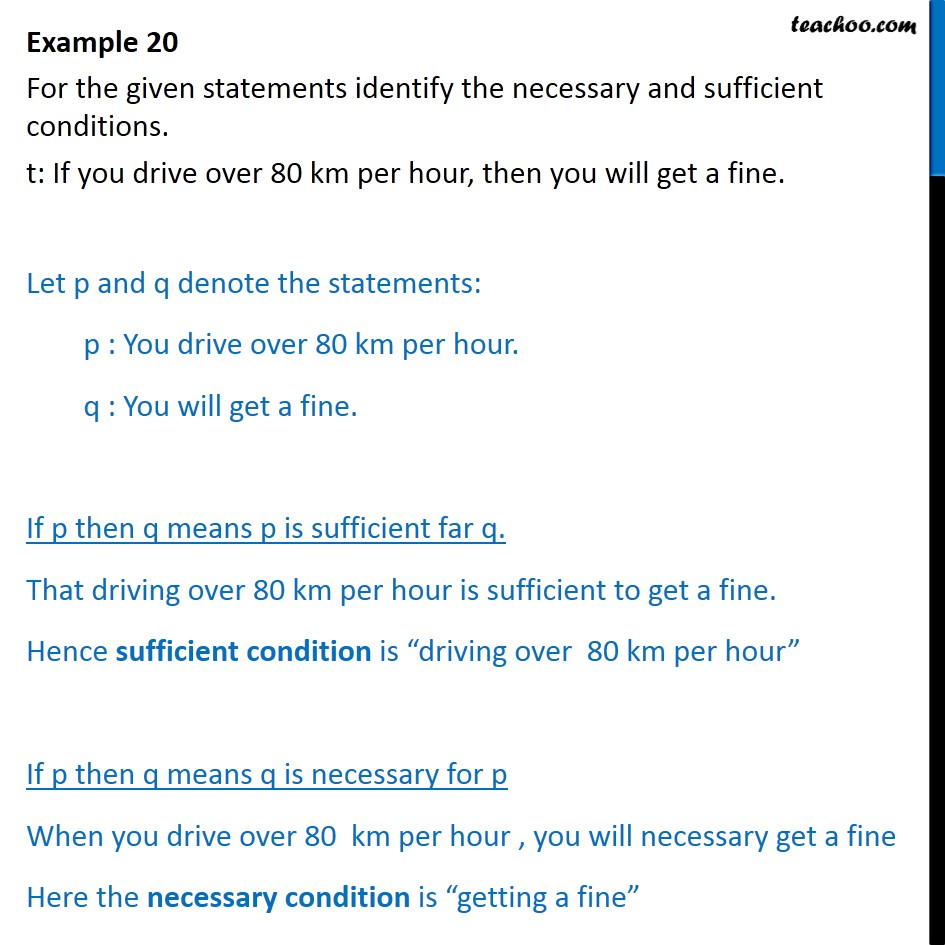Examples

Mathematical Reasoning
Serial order wiseLearn in your speed, with individual attention - Teachoo Maths 1-on-1 Class

### Transcript

Example 20 For the given statements identify the necessary and sufficient conditions. t: If you drive over 80 km per hour, then you will get a fine. Let p and q denote the statements: p : You drive over 80 km per hour. q : You will get a fine. If p then q means p is sufficient far q. That driving over 80 km per hour is sufficient to get a fine. Hence sufficient condition is driving over 80 km per hour If p then q means q is necessary for p When you drive over 80 km per hour , you will necessary get a fine Here the necessary condition is getting a fine